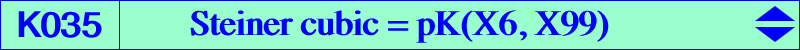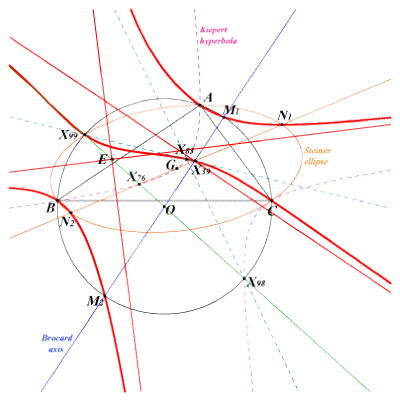X(1), X(39), X(83), X(99), X(512), X(1018), X(1019), X(1379), X(1380) excenters two intersections M1 and M2 of X(3)X(6) and the circumcircle two intersections N1 and N2 of X(2)X(39) and the Steiner ellipseLet P be a point in the triangle plane. Denote by Q and R its isogonal and isotomic conjugates, by QaQbQc and RaRbRc the cevian triangles of Q and R, by Ta, Tb, Tc the midpoints of QaRa, QbRb, QcRc. The triangles ABC and TaTbTc are perspective if and only if P lies on the Steiner cubic. This cubic is an isogonal pK with pivot the Steiner point X(99). One asymptote is perpendicular at X(39) to the Brocard axis. The two other are perpendicular and parallel to the asymptotes of the Kiepert hyperbola. They intersect at E midpoint of X(76)X(99). See another characterization at the end of CL027. See also the related cubics in table 17.